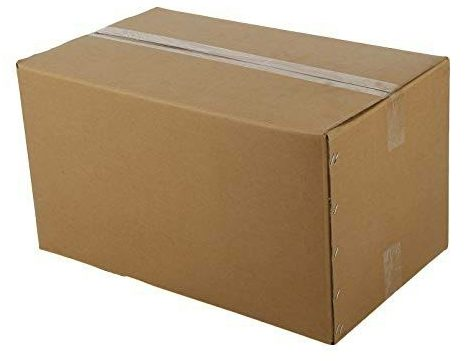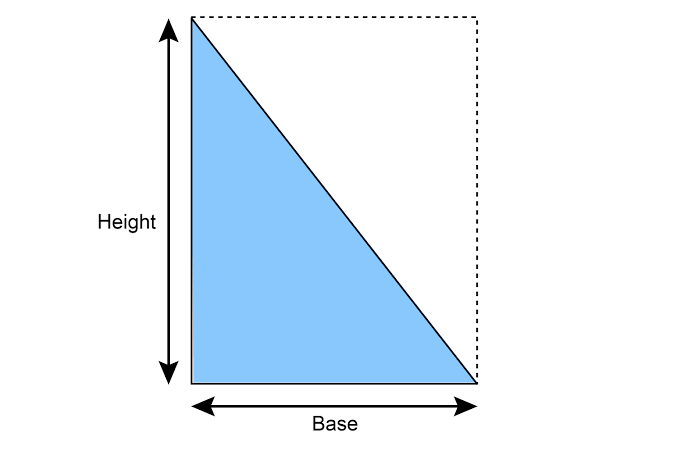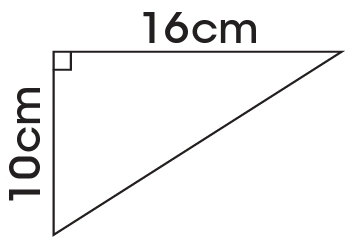# Area of Triangles (Primary 5)

MATHEMATICS

SECOND TERM

WEEK 7

PRIMARY 5

THEME – MEASUREMENTS AND GEOMETRY

PREVIOUS LESSON – Second Term Scheme of Work and Plan Lesson Notes for MATHEMATICS Week 1 to Week 12 Primary Schools

TOPIC – AREA OF RIGHT – ANGEL TRIANGLES

PERFORMANCE OBJECTIVES

By the end of the lesson, the pupils should have attained the following objectives (cognitive, affective and psychomotor) and should be able to calculate the area of a right angled triangle.

ENTRY BEHAVIOUR

Rectangle

INSTRUCTIONAL MATERIALS

The teacher will teach the lesson with the aid of cuboid, rectangle and triangle.

METHOD OF TEACHING – Choose a suitable and appropriate methods for the lessons.

Note – Irrespective of choosing methods of teaching, always introduce an activities that will arouse pupil’s interest or lead them to the lessons.

REFERENCE MATERIALS

1. Scheme of Work

2. 9 – Years Basic Education Curriculum

3. Course Book

4. All Relevant Material

5. Online Information

CONTENT OF THE LESSON

## LESSON ONE – INTRODUCTION

Pupil’s Activities 1 – Properties of Cuboid

Teacher’s Activities – Questions

1. The objects below is called ______________.2. What shape does represent in mathematics?

3. How many faces does the objects has?

4. Many corners or vertices does it have?

5. The object is used for ______________.

6. Mention 5 objects that can be packaged inside.

Note – Guides or leads the pupils where necessary.

Pupil’s Response

1. Carton or box

2. Cuboid

3. Six (6) faces

4. Eight vertices (corners)

5. Packaging

6. Indomie, wine, milk, television, radio, Maclean, chalk, marker, etc.

Teacher’s/Pupil’s Activities 2 – Introduction to Rectangle

Teacher’s Activities – Cut one of the faces of the carton. Asks pupils to draw and name it.

Pupil’s Response – Rectangle

Pupil’s Activities 3 – Lengths of Rectangle

Instructions/Questions

1. Measure each length of the rectangle.

2. Compare each length with another.

3. Use measurements to describe what rectangle means.

4. Mention 5 objects with rectangular face or shape.

Pupil’s Response

1. 10 cm, 7 cm, 10 cm and 7cm.

2. Two of the lengths are equal to each other.

3. Rectangle is a shape with 4 length. Two of its lengths are equal to another.

4. Board, sheet of paper, door, wall or floor, top of desk, etc.

Teacher’s remark – A rectangle is a 4 sided with opposite parallel lengths are equal length. It is one of the group called quadrilateral.

## LESSON TWO – RECTANGLE AND TRIANGLE

Pupil’s Activities 1 – Measure the Lengths of RectangleTeacher’s remark – The length and Breadth of rectangle are 7 cm and 3 cm.

Pupil’s Activities 2 – Area of Rectangle

Find the area of rectangle in activities 1 by multiplying length by Breadth.

= 7 cm x 3 cm

= 21 cm²

Pupil’s Activities 3 – Cutting Out A Right Angle Triangle

Instructions

1. Draw a diagonal and cut out the rectangle.

2. Then cut along the diagonal.

3. What shape did you cut out.Teacher’s remark – Two right angles are made up of a rectangle.

Teacher’s/Pupil’s Activities 2 – Area of Triangles

Draw and find the area of a rectangle of length 8 cm and breadth 5 cm.Area = 5 cm x 8 cm

= 40 cm²

Teacher’s question – If the area of the rectangle is 40 cm², what is the area of triangle if the rectangle is cut through the diagonal?Expected pupil’s response – The area of triangle is half of the rectangle. That’s, 40 cm² ÷  2 = 20 cm²

Pupil’s Activities 2 – Class Exercise/Take Home

Draw and find the area of rectangle whose

1. Length 12 cm and breadth 3 cm.

2. Length 15 cm and breadth 6 cm.

3. Length 4 cm and breadth 8 cm.

Then, find the area of triangle if the rectangle is cut through the diagonal.

## LESSON THREE – AREA OF TRIANGLES (½ b x h)

Teacher’s/Pupil’s Activities 1 – Area of TrianglesArea of rectangle = Length x Breadth

= L x B

Area of Triangle = ½ x L x B

= ½ x B x H  or ½bh

Where B stands for the base of the length and H is the breadth.Pupil’s Activities 2 – Application of ½bh

Find the areas of the triangle below,Area of Triangle = ½bh,

Where b = 8 cm and h = 6 cm

Therefore,

A = ½ x 8 cm x 6 cm

= 4 cm x 6 cm (8 cm ÷ 2 = 4 cm)

= 24 cm²

Pupil’s Activities 3 – Class Exercise/Take Home

Find the areas of triangles which have these sides,

1. Base is 4 cm and height is 5 cm.

2. Base is 12 m and height is 6 m.

4.## LESSON FOUR – QUANTITATIVE REASONING

As stated in the quantitative book or textbook.

PRESENTATION

To deliver the lesson, the teacher adopts the following steps:

1. To introduce the lesson, the teacher revises the previous lesson. Based on this, he/she asks the pupils some questions;

2. Guides pupils to divide a rectangle into two halves along its diagonal to form two equal right angled triangles.

3. Guides pupils to derive the formula for area of right angled triangles i.e. 1⁄2 of area of rectangle of 1⁄2 (base × heights).

4. Guides pupils to calculate the area of the right angled triangle.

Pupil’s Activities – Derive and use formula to calculate the area of a right angled triangle.

CONCLUSION

To conclude the lesson for the week, the teacher revises the entire lesson and links it to the following week’s lesson.

LESSON EVALUATION

Pupils to find the area of a given right angled triangle.